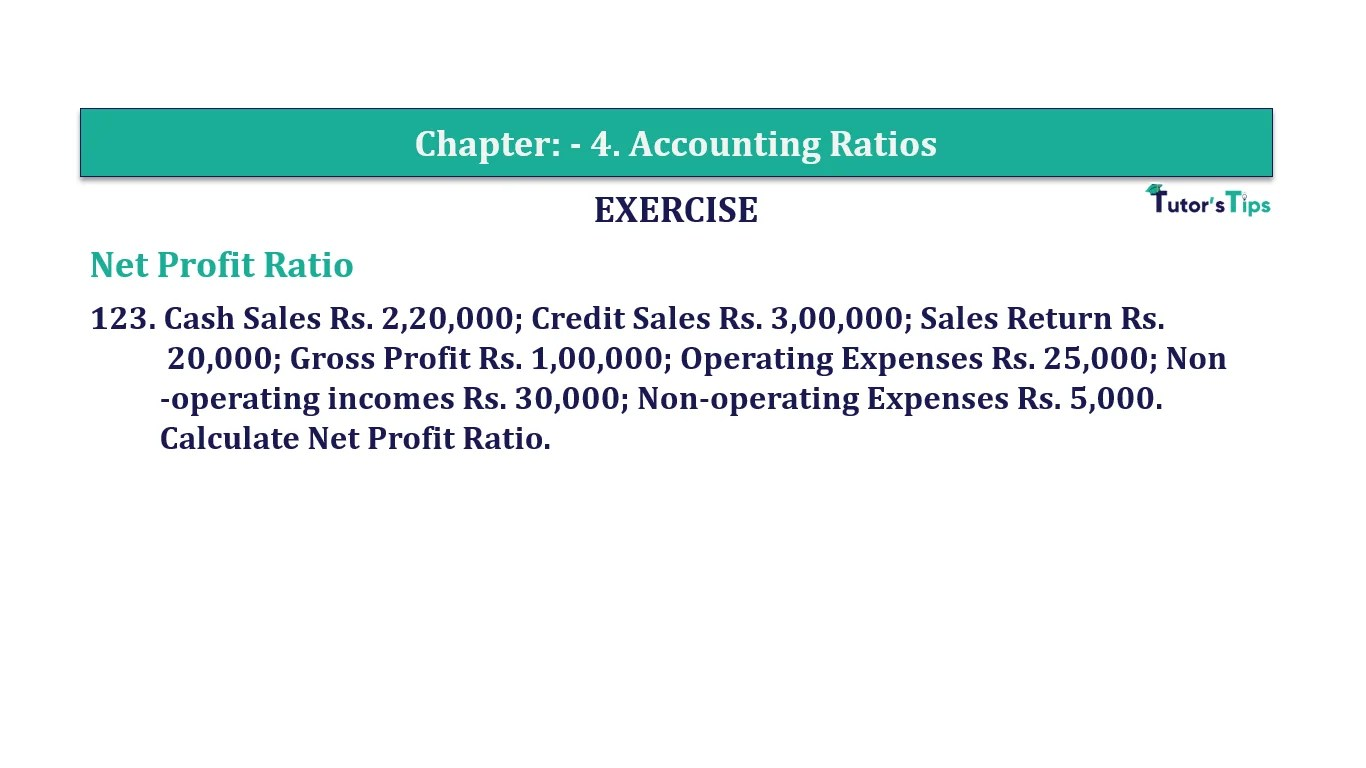# Question 123 Chapter 4 of +2-B – T.S. Grewal 12 ClassQuestion No. 123- Chapter No.4 - T.S. Grewal +2 Book Part B

Question 123 Chapter 4 of +2-B

Operating Ratio

123. Cash Sales Rs. 2,20,000; Credit Sales Rs. 3,00,000; Sales Return Rs.
20,000; Gross Profit Rs. 1,00,000; Operating Expenses Rs. 25,000; Non
-operating incomes Rs. 30,000; Non-operating Expenses Rs. 5,000.
Calculate Net Profit Ratio.

### The solution of Question 123 Chapter 4 of +2-B: –

 Net Sales = Cash Sales + Credit Sales – Sales Return = Rs. 2,20,000 + Rs. 3,00,000 – Rs. 20,000 = Rs. 5,00,000 Net Profit = Gross Profit – Operating Expenses – Non-operating Expenses + Non-operating Incomes = Rs. 1,00,000 – Rs. 25,000 – Rs. 5,000 + Rs. 30,000 = Rs. 1,00,000

 Operating Ratio = Operating Cost X 100 Net Sales
 Operating Ratio = Rs. 1,00,000 X 100 Rs. 5,00,000 = 20%

Balance Sheet: Meaning, Format & Examples

Comment if you have any question.

Also, Check out the solved question of previous Chapters: –